# Best Tensor Calculus Books 2023 | Enrich Your Mathematics Knowledge

The language of tensors, originally championed by Einstein, is as fundamental as the languages of calculus and linear algebra. Tensors are extensively used in physics as well as image analytics and subspace de-compositions.

Also, the study of mathematics and engineering remains incomplete without the study of tensor algebra. If you want to learn tensor algebra from the very beginning to advanced level. Below are the best tensor calculus books 2023 that will be the best choice for you.

## 1. Vector and Tensor Analysis (Dover Books on Mathematics)

An outstanding introduction to tensor analysis for physics and engineering students, this text admirably covers the expected topics in a careful step-by-step manner. In addition to the standard vector analysis of Gibbs, including dyadic or tensors of valence two, the treatment also supplies an introduction to the algebra of motors. The entire theory is illustrated by many significant applications. Surface geometry and hydrodynamics are treated at length in separate chapters. Nearly all of the important results are formulated as theorems, in which the essential conditions are explicitly stated. Each chapter concludes with a selection of problems that develop students' technical skills and introduce new and important applications. The material may be adapted for short courses in either vector analysis or tensor analysis.

## 2. What Are Tensors Exactly?

Tensors have numerous applications in physics and engineering. There is often a fuzzy haze surrounding the concept of tensor that puzzles many students. The old-fashioned definition is difficult to understand because it is not rigorous; the modern definitions are difficult to understand because they are rigorous but at a cost of being more abstract and less intuitive. The goal of this book is to elucidate the concepts in an intuitive way but without loss of rigor, to help students gain deeper understanding. As a result, they will not need to recite those definitions in a parrot-like manner any more. This volume answers common questions and corrects many misconceptions about tensors. A large number of illuminating illustrations helps the reader to understand the concepts more easily. This unique reference text will benefit researchers, professionals, academics, graduate students and undergraduate students.

## 3. Introduction to Tensor Analysis and the Calculus of Moving Surfaces

A complete textbook designed for advanced undergraduate and graduate students. The discussion of the calculus of moving surfaces is extended in this book. It provides you with an introduction to tensor analysis. It's very easy to read all about tensor analysis. Not much theory but enough discussion to get you to the point of doing some practical calculations.

What you'll learn-

• Differential geometry on manifolds
• Shape optimization
• Dynamic fluid film equations.
• Discussion of the interplay between algebra and geometry
• Fundamental geometry
• Various exciting applications
• Boundary perturbation of boundary value problems
• Geodesic equations
• Gauss-Bonnet theorem.

## 4. Schaums Outline of Tensor Calculus (Schaum's Outlines)

The book was published by famous Schaum's outline series. Written by the renowned expert and teachers. The main features of this book are helpful solved problems and step by step details on all solved problem.

What you'll learn-

• Around 300 solved problem
• Discussion about all course fundamentals
• Effective problem solving techniques
• Details on all major problems of tensor calculus

## 5. Tensor Analysis on Manifolds (Dover Books on Mathematics)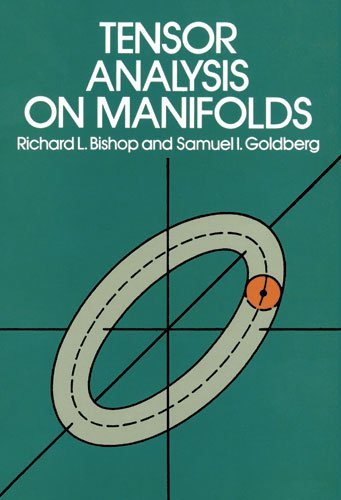Author: Richard L. Bishop,Samuel I. Goldberg
Published at: 01/12/1980
ISBN: 0486640396

This book introduces you to the essentials of modern vector, tensor analysis. No previous knowledge is needed. The topics are clearly arranged that you can easily find all the topics at a glance. Its essentials for the students of engineering and physics to learn about nature and applications of vectors and tensor analysis on manifolds

What you'll learn-

• Tensor analysis function-theoretical and algebraic aspects
• Vector analysis
• integration theory
• The special structure of tensor analysis
• Clarify the ideas of classical mechanics
• Numerous example and problems

## 6. Tensor Calculus Made Simple

A complete guide for tensor analysis. The method of presentation of ideas and techniques on tensor analysis makes it more preferable for students and researchers. You can use it as your reference book for an introductory course on tensor algebra.

What you'll learn-

• Fundamental equations of a surface
• General coordinate system
• Graphical illustrations of every problem
• Applications of tensor calculus
• Numerous problem and step by step solutions.

## 7. Vectors, Tensors and the Basic Equations of Fluid Mechanics (Dover Books on Mathematics)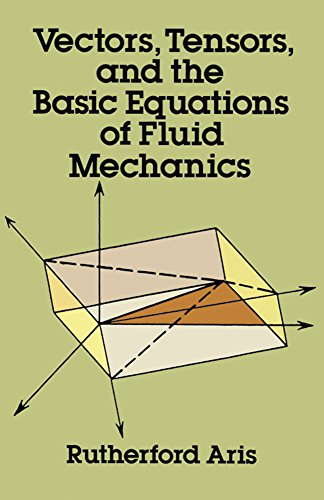Author: Rutherford Aris,Mathematics
Published at: 01/01/1990
ISBN: 0486661105

This book introduces you to the essentials of modern vector, tensor, and fluid mechanics. No previous knowledge is needed. The topics are clearly arranged that you can easily find all the topics at a glance. Its essentials for the students of engineering and physics to learn about nature and applications of vectors and tensor.

What you'll learn-

• Calculus of partial differentiation and multiple integrations
• Calculus of Cartesian vectors and tensors
• Equations of motion and energy in cartesian coordinates
• Equations of fluid flow in Euclidean space.
• Equations of surface flow
• Equations for reacting fluids
• 3-dimensional coordinate geometry
• Details on fluid motion and stress in fluids

## 8. Applications of Tensor Analysis (Dover Books on Mathematics)

This amazing book helps you to learn the fundamental ideas and the notation of tensor theory. It covers the introduction of the theory of differentiation of tensors and applied mathematics with dynamics, electricity, elasticity, and hydrodynamics.
What you'll learn-

• Algebraic preliminaries
• Algebraic geometry
• Differential geometry
• Fundamental equations of a surface
• Dynamics of rigid bodies, electricity, and magnetism
• Mechanics of continuous media
• The special theory of relativity

## 9. The Absolute Differential Calculus (Calculus of Tensors) (Dover Books on Mathematics)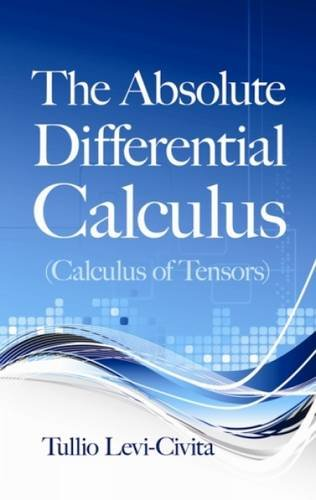Author: Tullio Levi-Civita
Published at: 17/04/2013
ISBN: 0486634019
This book was written by famous mathematicians of the twentieth century. The mathematical background is needed for understanding the tensor calculus. It provides introductory theories and basic discussion of fundamental quadratic forms. And it gives you details on absolute differential calculus and explores physical applications
What you'll learn-
• Functional determinants and matrics
• Linear partial determinants
• Algebraic functions
• Curvature-related Riemann's symbols and properties
• Intrinsic geometry
• Gravitational equations and general relativity

## 10. Principles of Tensor Calculus: Tensor Calculus

This well-decorated book helps you to clarify your basics of tensor calculus. No previous knowledge is needed. The topics are clearly arranged that you can easily find all the topics at a glance. It provides various techniques of the tensor equation and solved any kind of calculation problems easily

What you'll learn-

• Cartesian approaches
• General co-ordinate system
• Graphical illustrations of every problem
• Basics of tensor calculus

## 11. Manifolds, Tensors, and Forms: An Introduction for Mathematicians and Physicists

This book introduces you to the essentials of modern differential geometry and topology. Clear definitions and step by step details make it perfect for undergraduate and graduate students. It discusses the very basics of manifolds and tensor.

What you'll learn-

• Multi-linear algebra
• Homology
• Differentiation and integration on manifolds
• Cohomology
• Pseudo-Riemannian geometry
• 250 details exercise
• Classical mechanics
• General relativity and gauge theory
• Electromagnetism

## 12. A Student's Guide to Vectors and Tensors

The most powerful problem-solving tools on vector and tensor with applications of mechanics and electromagnetism. Its essentials for the students of engineering and physics to learn about nature and applications of vectors and tensor. This book provides all basic and practical applications of tensor for engineering students.

What you'll learn-

• Maxwell's equations
• Vector and vector calculus
• Matrices and their algebra
• Interactive solutions to all problems
• Graphical explanations of tensor

## 13. Essential Calculus Skills Practice Workbook with Full Solutions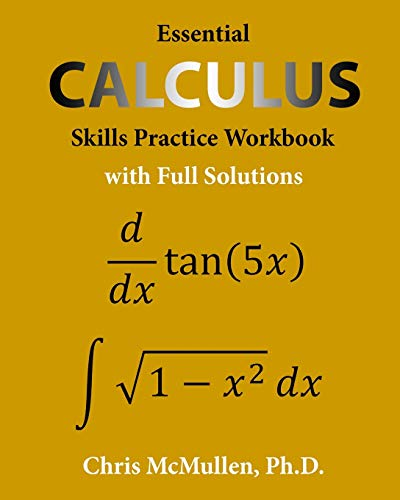Author: McMullen, Chris
Published at: 16/08/2018
ISBN: 1941691242

The author, Chris McMullen, PhD, has over twenty years of experience teaching math skills to physics students. He prepared this comprehensive workbook (with full solutions to every problem) to share his strategies for mastering calculus. This workbook covers a variety of essential calculus skills, including:

derivatives of polynomials, trig functions, exponentials, and logarithms the chain rule, product rule, and quotient rule second derivatives how to find the extreme values of a function limits, including l’Hopital’s rule antiderivatives of polynomials, trig functions, exponentials, and logarithms definite and indefinite integrals techniques of integration, including substitution, trig sub, and integration by parts multiple integrals.

## 14. Solutions of Exercises of Tensor Calculus Made Simple

This book contains the detailed solutions of all the exercises of my book: Tensor Calculus Made Simple. The solutions are generally very detailed and hence they are supposed to provide some sort of revision for the subject topic.

## 15. Solutions of Exercises of Principles of Tensor Calculus

This book contains the solutions of all the exercises of my book: Principles of Tensor Calculus. These solutions are sufficiently simplified and detailed for the benefit of readers of all levels particularly those at introductory levels.

## 16. Tensor Algebra and Tensor Analysis for Engineers: With Applications to Continuum Mechanics (Mathematical Engineering)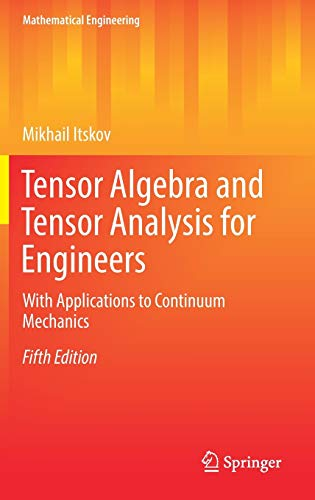Author: Itskov, Mikhail
Published at: 27/09/2018
ISBN: 3319988050

This is the fifth and revised edition of a well-received textbook that aims at bridging the gap between the engineering course of tensor algebra on the one hand and the mathematical course of classical linear algebra on the other hand. In accordance with the contemporary way of scientific publication, a modern absolute tensor notation is preferred throughout. The book provides a comprehensible exposition of the fundamental mathematical concepts of tensor calculus and enriches the presented material with many illustrative examples. As such, this new edition also discusses such modern topics of solid mechanics as electro- and magnetoelasticity. In addition, the book also includes advanced chapters dealing with recent developments in the theory of isotropic and anisotropic tensor functions and their applications to continuum mechanics. Hence, this textbook addresses graduate students as well as scientists working in this field and in particular dealing with multi-physical problems. In each chapter numerous exercises are included, allowing for self-study and intense practice. Solutions to the exercises are also provided.

Thanks for reading this post. If you have any opinion don't hesitate to comment here. Also please subscribe our newsletter to get more updates.